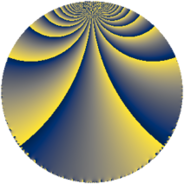# Properties

 Label 75.3.kLevel $75$ Weight $3$ Character orbit 75.k Rep. character $\chi_{75}(13,\cdot)$ Character field $\Q(\zeta_{20})$ Dimension $80$ Newform subspaces $1$ Sturm bound $30$ Trace bound $0$

# Learn more

## Defining parameters

 Level: $$N$$ $$=$$ $$75 = 3 \cdot 5^{2}$$ Weight: $$k$$ $$=$$ $$3$$ Character orbit: $$[\chi]$$ $$=$$ 75.k (of order $$20$$ and degree $$8$$) Character conductor: $$\operatorname{cond}(\chi)$$ $$=$$ $$25$$ Character field: $$\Q(\zeta_{20})$$ Newform subspaces: $$1$$ Sturm bound: $$30$$ Trace bound: $$0$$

## Dimensions

The following table gives the dimensions of various subspaces of $$M_{3}(75, [\chi])$$.

Total New Old
Modular forms 176 80 96
Cusp forms 144 80 64
Eisenstein series 32 0 32

## Trace form

 $$80q + 4q^{2} + 4q^{5} - 4q^{7} - 12q^{8} + O(q^{10})$$ $$80q + 4q^{2} + 4q^{5} - 4q^{7} - 12q^{8} - 4q^{10} - 24q^{12} + 32q^{13} - 24q^{15} + 80q^{16} - 100q^{17} - 48q^{18} - 100q^{19} - 244q^{20} - 100q^{22} - 96q^{23} - 16q^{25} - 40q^{26} + 196q^{28} + 200q^{29} + 264q^{30} + 636q^{32} + 216q^{33} + 100q^{34} + 260q^{35} - 120q^{36} - 184q^{37} - 564q^{38} - 948q^{40} + 160q^{41} - 12q^{42} - 472q^{43} - 700q^{44} - 36q^{45} - 288q^{47} - 48q^{48} + 16q^{50} + 620q^{52} + 304q^{53} + 604q^{55} + 72q^{57} + 1272q^{58} + 800q^{59} + 84q^{60} - 240q^{61} + 1212q^{62} - 12q^{63} + 100q^{64} + 272q^{65} - 80q^{67} + 104q^{68} - 260q^{70} + 36q^{72} - 116q^{73} - 24q^{75} - 88q^{77} - 120q^{78} + 200q^{79} - 164q^{80} + 180q^{81} - 168q^{82} - 1264q^{83} - 1200q^{84} - 212q^{85} - 876q^{87} - 212q^{88} - 1500q^{89} - 444q^{90} - 1504q^{92} - 648q^{93} - 200q^{94} - 784q^{95} + 60q^{96} - 260q^{97} - 92q^{98} + O(q^{100})$$

## Decomposition of $$S_{3}^{\mathrm{new}}(75, [\chi])$$ into newform subspaces

Label Dim. $$A$$ Field CM Traces $q$-expansion
$$a_2$$ $$a_3$$ $$a_5$$ $$a_7$$
75.3.k.a $$80$$ $$2.044$$ None $$4$$ $$0$$ $$4$$ $$-4$$

## Decomposition of $$S_{3}^{\mathrm{old}}(75, [\chi])$$ into lower level spaces

$$S_{3}^{\mathrm{old}}(75, [\chi]) \cong$$ $$S_{3}^{\mathrm{new}}(25, [\chi])$$$$^{\oplus 2}$$